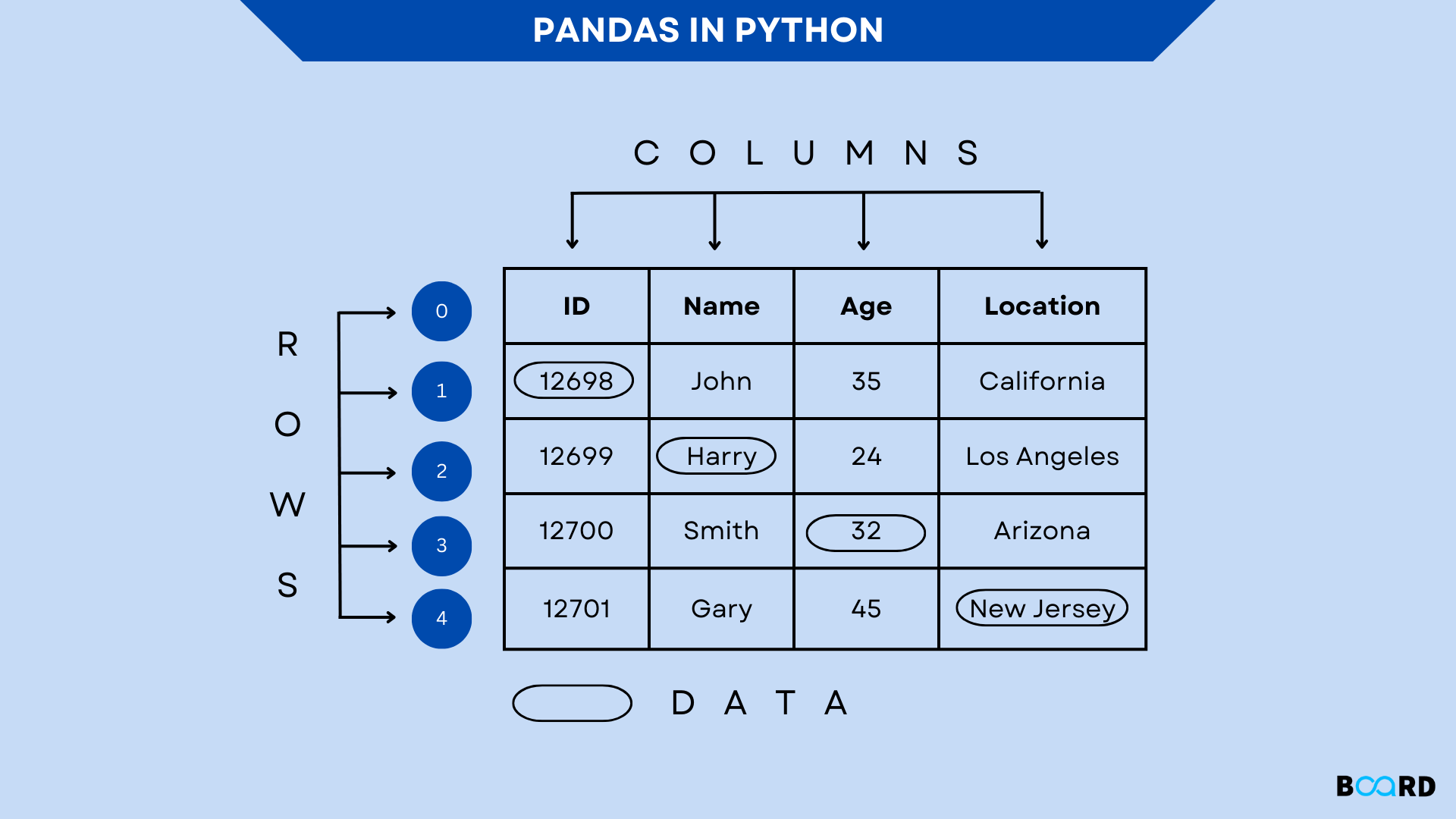# What is pandas in python - Pandas Introduction## Introduction

The most well-known open-source library for the python programming language is called pandas, and machine learning and data science applications frequently use pandas. It is built on top of Numpy, a well-known package that supports multi-dimensional arrays and offers scientific computing in Python. It is created by Wes McKinney; find out what other projects he is working on on his GitHub page. The first two data structures that Pandas supports are listed below.

• pandas Series
• pandas DataFrame
• pandas Index

### Install Pandas using Python pip Command

Third-party packages from PyPI are installed using the Python package manager pip. You may install, remove, upgrade, or downgrade any Python library that is listed in the Python Package Index using the pip tool. We should utilize PyPI (Python Package Index) to install the most recent version of pandas on Windows as it contains the pandas package.

 # Install pandas using pippip install pandas(or)pip3 install pandas

### Run Pandas From Command Line

If you installed Anaconda, open the command prompt in Python or the Anaconda command line and type the following commands to obtain the version of pandas. For more information, refer to the links on the pandas tutorial's left side.

 >>> import pandas as pd>>> pd.__version__'1.3.2'

Learn how to construct a Series using examples in this section of the pandas tutorial. A Series is a one-dimensional array that may store a variety of data types (integer, string, float, python objects, etc.). Using the series() function, we can quickly transform the list, tuple, and dictionary into Series. The row labels of Series are referred to as the index in pandas Series. The Series can only have one column; it cannot have more than one. You can convert a List, NumPy Array, or Dict into a pandas Series.

## Pandas.series() Constructor

The syntax for creating Series objects using the pandas Series Constructor is listed below.

 # Pandas Series Constructor SyntaxPandas.series(data,index,dtype,copy)
• data: Ndarray, list, and constants are present in the data.
• Index: The index has to be hashable and distinct. if no index is provided, np.arrange(n).
• dtype: Another data type is dtype.
• copy: It is employed to copy data. Ndarray, list, and constants are present in the data.

### Create Pandas Series

Array, list, dict, and existing DataFrames can all be used to create pandas Series.

#### Creating Series from NumPy Array

 # Create Series from arrayimport pandas as pd import numpy as npdata = np.array(['python','php','java'])series = pd.Series(data)print (series)

#### Creating Series from Dict

 # Create a Dict from a inputdata = {'Courses' :"pandas", 'Fees' : 20000, 'Duration' : "30days"}s2 = pd.Series(data)print (s2)

#### Creating Series from List

 #Creating DataFrame from Listdata = ['python','php','java']s2 = pd.Series(data, index=['r1', 'r2','r3'])print(s2)
write your code here: Coding Playground

## Pandas DataFrame

I have a tutorial specifically for Python Pandas DataFrame, so I won't go into too much detail here. DataFrame is a tabular data structure with labeled axes, rows, and columns that is two-dimensional, immutable, and heterogeneous. There are three main parts to a pandas Dataframe: data, rows, and columns.

### DataFrame Features

• Support for named rows and columns in DataFrames (you can also provide names to rows)
• The Pandas DataFrame size can be changed.
• Supports heterogeneous data collections.
• DataFrame axes with labels (rows and columns).
• Can operate mathematically on both rows and columns.
• Supporting the reading of flat files like CSV, Excel, and JSON as well as the handling of missing data in SQL tables.

## Pandas Series vs DataFrame?

• As I said above, the difference between the DataFrame and the Panda Series is that the latter is a two-dimensional labeled data structure with columns that may be of several data kinds.
• The Panda Series is a one-dimensional labeled array of the same data type.
• Each column of data in a DataFrame is represented by a pandas Series.
• Series cannot have a column name, however DataFrame columns can have names or labels.
• Additionally, a DataFrame may be transformed to a single or several Series, and vice versa.
• For further information and examples on DataFrame, see the pandas DataFrame Tutorial.